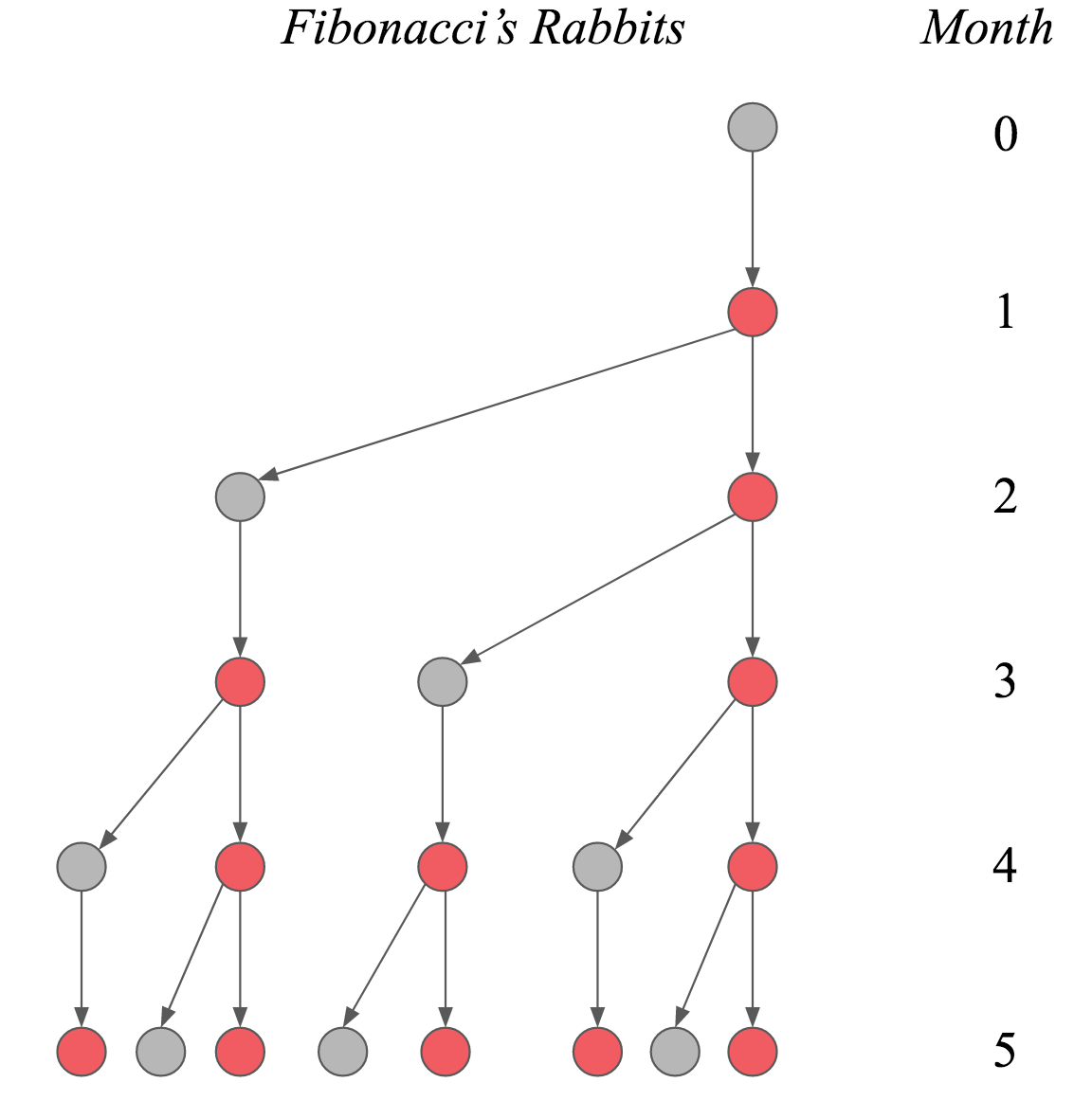# The Fibonacci sequence and linear algebra

Leonardo Bonacci, better known as Fibonacci, has influenced our lives profoundly. At the beginning of the $13^{th}$ century, he introduced the Hindu-Arabic numeral system to Europe. Instead of the Roman numbers, where I stands for one, V for five, X for ten, and so on, the Hindu-Arabic numeral system uses position to index magnitude. This leads to much shorter expressions for large numbers.

While the history of the numerical system is fascinating, this blog post will look at what Fibonacci is arguably most well known for: the Fibonacci sequence. In particular, we will use ideas from linear algebra to come up with a closed-form expression of the $n^{th}$ Fibonacci number. On our journey to get there, we will also gain some insights about recursion in R.

In Liber Abaci, Fibonacci poses the following question (paraphrasing):

Suppose we have two newly-born rabbits, one female and one male. Suppose these rabbits produce another pair of female and male rabbits after one month. These newly-born rabbits will, in turn, also mate after one month, producing another pair, and so on. Rabbits never die. How many pairs of rabbits exist after one year?

The Figure below illustrates this process. Every point denotes on rabbit pair over time. To indicate that every newborn rabbit pair needs to wait one month before producing new rabbits, rabbits that are not fertile yet are coloured in grey, while rabbits ready to procreate are coloured in red.We can derive a linear recurrence relation that describes the Fibonacci sequence. In particular, note that rabbits never die. Thus, at time point $n$, all rabbits from time point $n – 1$ carry over. Additionally, we know that every fertile rabbit pair will produce a new rabbit pair. However, they have to wait one month, so that the amount of fertile rabbits equals the amount of rabbits at time point $n – 2$. Resultingly, the Fibonacci sequence {$F_n$}$_{n=1}^{\infty}$ is:

for $n \geq 3$ and $F_1 = F_2 = 1$. Before we derive a closed-form expression that computes the $n^{th}$ Fibonacci number directly, in the next section, we play around with alternative, more straightforward solutions in R.

We can write a wholly inefficient, but beautiful program to compute the $n^{th}$ Fibonacci number: